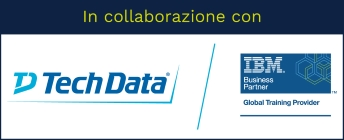Stai utilizzando un browser vecchio, che non rispetta gli standard web. Ti consigliamo di cambiarlo, perché questo sito potrebbe non funzionare correttamente.

# Introduction to Time Series Analysis Using IBM SPSS Modeler (v18.1.1)

## IBM0A028G

This course gets you up and running with a set of procedures for analyzing time series data. Learn how to forecast using a variety of models, including regression, exponential smoothing, and ARIMA, which take into account different combinations of trend and seasonality. The Expert Modeler features will be covered, which is designed to automatically select the best fitting exponential smoothing or ARIMA model, but you will also learn how to specify your own custom models, and also how to identify ARIMA models yourself using a variety of diagnostic tools such as time plots and autocorrelation plots.

### Argomenti

1: Introduction to time series analysis

• Explain what a time series analysis is
• Describe how time series models work
• Demonstrate the main principles behind a time series forecasting model
2: Automatic forecasting with the Expert Modeler
• Examine fit and error
• Examine unexplained variation
• Examine how the Expert Modeler chooses the best fitting time series model
3: Measuring model performance
• Discuss various ways to evaluate model performance
• Evaluate model performance of an ARIMA model
• Test a model using a holdout sample
4: Time series regression
• Use regression to fit a model with trend, seasonality and predictors
• Handling predictors in time series analysis
• Detect and adjust the model for autocorrelation
• Use a regression model to forecast future values
5: Exponential smoothing models
• Types of exponential smoothing models
• Create a custom exponential smoothing model
• Forecast future values with exponential smoothing
• Validate an exponential smoothing model with future data
6: ARIMA modeling
• Explain what ARIMA is
• Learn how to identify ARIMA model types
• Use sequence charts and autocorrelation plots to manually identify an ARIMA model that fits the data
• Check your results with the Expert Modeler

### Obiettivi

Introduction to time series analysis

Automatic forecasting with the Expert Modeler

Measuring model performance

Time series regression

Exponential smoothing models

ARIMA modeling

## Prezzo di listino

700,00 EUR + IVA per partecipante

• 8 ore
• 1 giorno

## Prerequisiti

• Familiarity with the IBM SPSS Modeler environment (creating, editing, opening, and saving streams).
• General knowledge of regression analysis is recommended but not required

# Erogabile on-line e on-site

Tutti i nostri corsi sono erogabili anche in modalità on-line (con formazione a distanza), oppure on-site, sempre personalizzati secondo le esigenze.